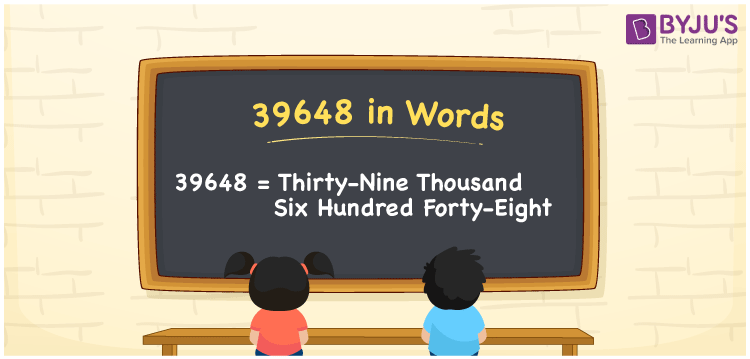39648 in words is Thirty-Nine Thousand Six Hundred Forty-eight. For example, if you spend Rs. 39648 in a month, you can write, “I have spent Rs. Thirty-Nine Thousand Six Hundred Forty-eight in a month”. In general, the number name of any number can be written using the ones, tens, hundreds, and thousands place of a number. Thus, the place value chart is useful for writing the number 39648 in words.

 39648 in words Thirty-Nine Thousand Six Hundred Forty-eight Thirty-Nine Thousand Six Hundred Forty-eight in Numbers 39648

## 39648 in English Words## How to Write 39648 in Words?

We can convert 39648 to words using a place value chart. This can be done as follows. The number 39648 has 5 digits, so let’s make a chart that shows the place value up to 5 digits.

 Ten thousands Thousands Hundreds Tens Ones 3 9 6 4 8

Thus, we can write the expanded form as:

3 × Ten thousand + 9 × Thousand + 6 × Hundred + 4 × Ten + 8 × One

= 3 × 10000 + 9 × 1000 + 6 × 100 + 4 × 10 + 8 × 1

= 39648

= Thirty-Nine Thousand Six Hundred Forty-eight

39648 is the natural number that is succeeded by 39647 and preceded by 39649.

39648 in words – Thirty-Nine Thousand Six Hundred Forty-eight

Is 39648 an odd number? – No.

Is 39648 an even number? – Yes

Is 39648 a perfect square number? – No

Is 39648 a perfect cube number? – No

Is 39648 a prime number? – No

Is 39648 a composite number? – Yes

## Frequently Asked Questions on 39648 in Words

Q1

### How to write 39648 in words?

39648 in English is written as “Thirty-Nine Thousand Six Hundred Forty-eight”.
Q2

### Is the number 39648 an odd number?

No, the number 39648 is not an odd number.
Q3

### Is 39648 a composite number?

Yes, 39648 is a composite number.